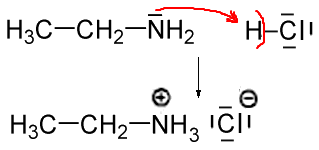Search:

# The basicity of ethanamine (ethylamine)

## A proton acceptor

### Experiment

The Erlenmeyer flask contains chlorhydric gas, a cotton swab is moistened with a solution of ethanamine:A white smoke (smoke = solid particles) ethylammonium chloride is produced. $CH_3CH_2NH_2 (g)$ $+$ $HCl (g)$ $\rightarrow$ $CH_3CH_2NH_3^+ Cl^-(s)$

### InterpretationBy its electron pair on the nitrogen, which is not strongly retained (N is weakly electronegative), the amine captures a hydrogen ion $H^+$: The amine is a proton acceptor and thus a base by defining Broenstedt!

## A weak base

### Experiment

A light bulb is used to study the conductivity of aqueous ethylamine:The current is weak, because there are some ions in solution, but very few compared to solutions of strong acids or strong bases . The reaction with water is limited to an equilibrium:

$CH_3CH_2NH_2 (g)$ $+$ $H_2O$ $\leftrightarrows$ $CH_3CH_2NH_3^+$ $+$ $OH^-$